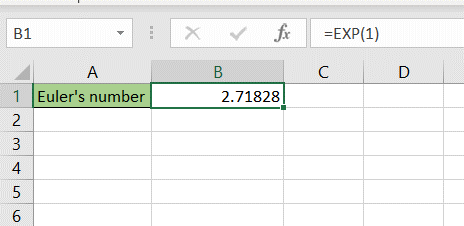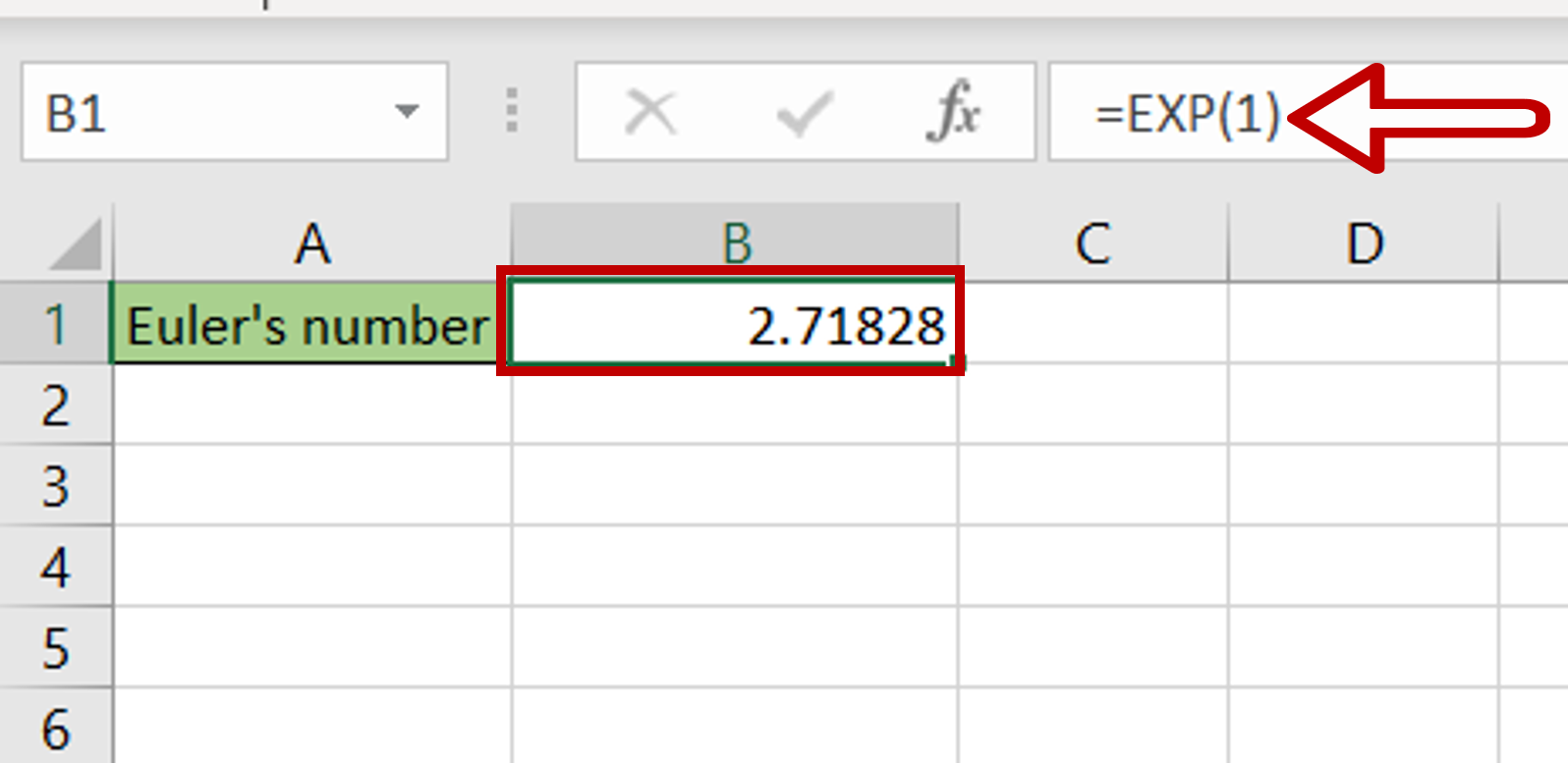# How to write e in Excel

You can watch a video tutorial here.The symbol ‘e’ stands for Euler’s number and is the base for natural logarithms. It is approximately 2.71828182845904. In Excel, to write e you can use the EXP() function. This will raise e to the power of the number. The syntax of EXP() is as follows:

– Syntax: EXP(number)
>number: the number that e is to be raised to

### Step 1 – Create the EXP() formula– Select the cell where e is to be displayed
– Type the formula:
=EXP(1)
– Using 1 as the power returns the number itself